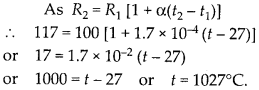Enlightened

# At room temperature (27.0°C) the resistance of a heating element is 100 O. What is the temperature of the element if the resistance is found to be 117 Ω, given that the temperature coefficient of the material of the resistor is 1.70 × 103 0C-1.

• 0

At room temperature (27.0°C) the resistance of a heating element is 100 O. What is the temperature of the element if the resistance is found to be 117 Ω, given that the temperature coefficient of the material of the resistor is 1.70 × 103 0C-1.

Share